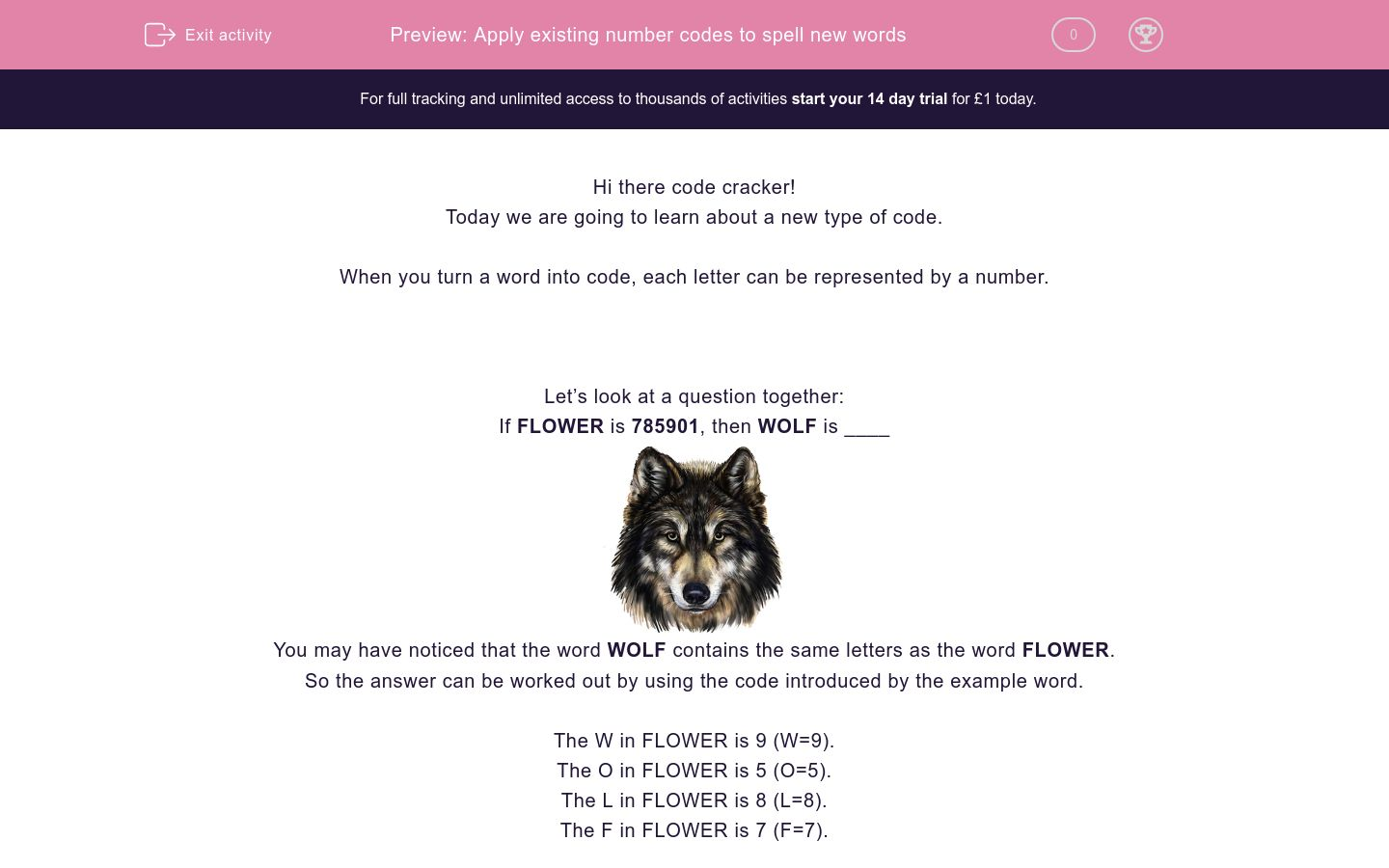# Apply existing number codes to spell new words

In this worksheet, students will turn words into number codes using words with similar codes as a base. It will develop their encoding and problem solving skills.Key stage:  KS 2

Curriculum topic:   Verbal Reasoning

Curriculum subtopic:   Number Code Breaking

Difficulty level:#### Worksheet Overview

Hi there code cracker!

Today we are going to learn about a new type of code.

When you turn a word into code, each letter can be represented by a number.

Let’s look at a question together:

If FLOWER is 785901, then WOLF is ____You may have noticed that the word WOLF contains the same letters as the word FLOWER.

So the answer can be worked out by using the code introduced by the example word.

The W in FLOWER is 9 (W=9).

The O in FLOWER is 5 (O=5).

The L in FLOWER is 8 (L=8).

The F in FLOWER is 7 (F=7).

So WOLF is 9587 in code.

Let’s try another:

If the code for DINOSAUR is 51372894, then AROUND is ____

The word DINOSAUR contains the same letters as AROUND, so we can use this to work out the new code.

So the code for AROUND is 847935.

In this activity, you will be a code cracker and change words into number codes. Make a note of the number that matches each letter; you may want to create your own grid to help with this.

It can be really helpful to write the word out and then write the corresponding numbers above each letter.  Give it a go!

Let’s get started!

### What is EdPlace?

We're your National Curriculum aligned online education content provider helping each child succeed in English, maths and science from year 1 to GCSE. With an EdPlace account you’ll be able to track and measure progress, helping each child achieve their best. We build confidence and attainment by personalising each child’s learning at a level that suits them.

Get started